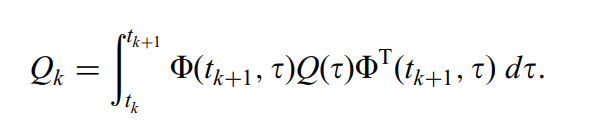# Continuous-time process noise

I’m implementing a Kalman Filter Estimator and I’d like to define my process noise matrix in continuous time. The following equation is out of Grewal and Andrews:where Q(t) is the continuous time process noise, Qk is discrete time (which the filter needs) and Phi is the state transition matrix.

A couple of ideas I’ve had are:

• do a coarse Euler integration, but I can’t find a method that will allow me to just predict the Kalman Estimator to a future time without an observation?
• add additional equations to the numerical propagator, but that isn’t an option for the numericalpropagatorbuilder?

Is there a way that I can implement one of these methods, or is there an alternative that I can use?

You should not add the variational equations by yourself as they are already added automatically by the estimator. One possible way would be to change the visibility of `getErrorStateTransitionMatrix` in class `KalmanModel` from private to package protected and add the following public method to the class `KalmanEstimator`:

``````    /** Get the normalized error state transition matrix (STM) from previous point to current point.
* The STM contains the partial derivatives of current state with respect to previous state.
* The  STM is an mxm matrix where m is the size of the state vector.
* m = nbOrb + nbPropag + nbMeas
* @return the normalized error state transition matrix
*/
public RealMatrix getErrorStateTransitionMatrix() {
return processModel.getErrorStateTransitionMatrix();
}
``````

Then, if you manage to forward a reference to the estimator down to your `CovarianceMatrixProvider`, you will get access to a state transition matrix. I am not sure you will get the STM at the proper date, perhaps you will get the one from the previous step, you’ll have to check.

If this work for you, then open an issue on the forge so we add these changes in the next release.

After some thoughts, what I propose will not work because it would correspond to a discrete STM, and you need the continuous one in your integration.

So you really need to add your equation as an additional equation (the sub-integral part, that will be integrated automatically along the other equations). It is possible in the development version since a few weeks, as per merge request 25. This is available in the develop branch, which is not released yet.

This will be tricky as in your additional equation, you will need to get access to the additional states resulting from another additional equation (the instance of `PartialDerivativesEquations` automatically added by the estimator) in order to get the Φ matrix. There may be some calls scheduling issues (which additional equation is called first ?) and some access issues (does an additional equation see the state from previously called additional equations ?). Also the `PartialDerivativesEquations` is automatically added quite late, during the `KalmanEstimator` built, which needs the propagator builder. So if you need your equation to be located after the automatically added `PartialDerivativesEquations` in the list, you must create your propagator builder, then create the `KalmanEstimator` and only afterwards add your custom additional equation to the propagator builder.

Beware that the instance of `PartialDerivativesEquations` automatically added by the estimator is reset at the end of each estimation step, so you will need to re-add your own equation too at the end of each estimation step. In order to do so, you will also need to extend `KalmanModel`, override its `finalizeEstimation` method in order to first call the original one (calling `super.finalizeEstimation(observedMeasurement, estimate)`) and then re-add your equation. You will also need to extend `NumericalPropagatorBuilder` and override its `buildKalmanModel` method so it creates an instance of your extended Kalman model and not the original one.

This is a complex task, let us know it all this stuff works. If so, we may try to make it more straightforward.

Hi Luc. That is amazing!
Thank you very much for the comprehensive answer. It is going to take me some time to digest what you’ve written and a bit longer to code it up.
I’ll let you know how I go.